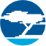# Traveo II Automotive Body Controller - FAQ – LIN - KBA232509Employee
Employee# 8. LIN

##### 8.1. I am unable to get proper baud rate for LIN. How is the LIN baud rate is calculated from the peripheral clock?

To set up the LIN clock, set the clock divider for the peripheral clock, which is being used for the LIN Component. The LIN baud rate is calculated by:

Baud Rate = (Peri Clock)/ (16* Divider value).

So, Divider value= (Peri Clock)/ (16* Baud Rate).

Here 16 is the oversampling factor that is fixed in Traveo II device. So, set the divider value correctly to get the appropriate baud rate. For instance, for a peripheral clock of 80 MHz, the required baud rate is 19.3 kbps; since you are using a 16-bit integer divider, the divider value is 260 (0x104). You need to set the value of the INT16_DIV of PERI_DIV_16_CTL_x register to divider value minus one. In this example, set the value of the INT16_DIV of PERI_DIV_16_CTL_x register to 259 (0x103) (260-1).

For more details, see the section Peripheral Clock Divider Configuration of the Architecture TRM.  For specific details on the divider configurations and the available divider resources, see the device datasheet.

304 Views